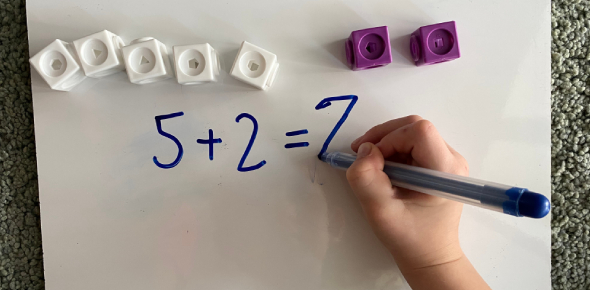# Word Problem Exam: MCQ Quiz!

10 Questions | Total Attempts: 51Settings.

• 1.
Divide:  235. 41 by 1000
• A.

2354.1

• B.

0.023541

• C.

0.23541

• D.

23541

• 2.
Divide: 0.768 by 1.6
• A.

48

• B.

4.8

• C.

0.4

• D.

0.48

• 3.
A vehicle covers a distance 0f 43.2 km in 2.4 liters of petrol. How much distance will travel in 1 liter of petrol?
• A.

20

• B.

0.18

• C.

1.8

• D.

18

• 4.
0.0038 * 100 = [Blank]
• 5.
The product of two decimals is 42.987. If one of them is 12.46, find the other.
• A.

3.45

• B.

34.5

• C.

345

• D.

0.345

• 6.
One meter of cloth costs Rs 152.50. What is the cost of 10.75 meters of cloth?
• A.

1639375

• B.

16.39375

• C.

1639.375

• D.

16393.75

• 7.
By how much should 59.71 be decreased to get 34.58?
• A.

24.13

• B.

26.13

• C.

25.12

• D.

25.13

• 8.
What is to be added to 36.85 to get 59.41?
• A.

22.57

• B.

22.56

• C.

23.56

• D.

21.56

• 9.
Write following fraction as decimal: (8/100)
• A.

0.8

• B.

800

• C.

0.08

• D.

0.008

• 10.
Find the value of 48.1 – 0.37
• A.

48.73

• B.

46.73

• C.

45.73

• D.

47.73

Related TopicsBack to top